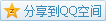\begin{aligned}\iint_{R_0}x\mathrm{d}A&=S_{R_0}x_0\\\iint_{R_1}x\mathrm{d}A&=S_{R_1}x_1\\\iint_{R_2}x\mathrm{d}A&=S_{R_2}x_2,\end{aligned}